# Chi-Square-Test-of-Independence– Excel and Google Sheets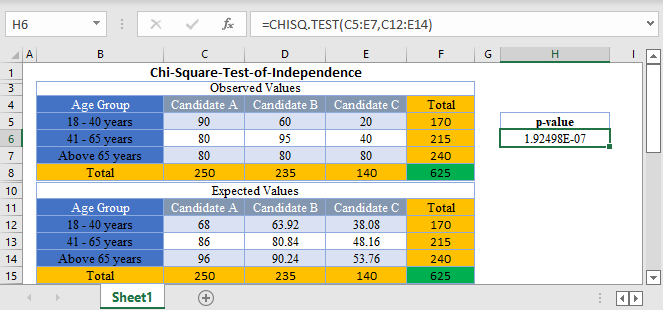Chi-Square (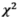) test of independence is a statistical test used to determine whether there is a relationship

(association) between two variables.

### Conditions for Chi-Square Test of Independence

• The data points should be frequencies or counts and not percentages or other forms of data.
• The categories of the variables should be mutually exclusive. That is, each data point can only belong to one category.
• The subject of study must be independent.
• The Expected Values should be at least 5.

### How to Perform Chi-Square Test of Independence in Excel

There are two ways to perform Chi-Square Tests in Excel:

1. The TEST Function, and
2. The INV.RT Function.

## CHISQ.TEST Function

The CHISQ.TEST Function returns the p-value of the set of data which is used to make a conclusion whether there is an association between the variables.

If the p-value is less than the significant level, it means that there is an association/relationship between the variables in the dataset.

### CHISQ.TEST Function Syntax

``=CHISQ.TEST(actual range, expected range)``

### How to Use CHISQ.TEST Function

Background: A political researcher wants to know if there is a relationship between the age distribution of voters and their preference for the three leading candidates for an election. He surveyed voters of different ages on their preferred candidates and presented the result in the table below. Test, at a 5% significant level, whether there is an association between the age distribution of voters and their choice of candidate.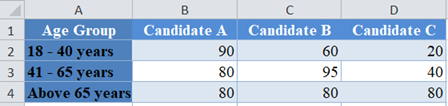First, calculate the total for each row and each column and calculate the grand total using the SUM Function.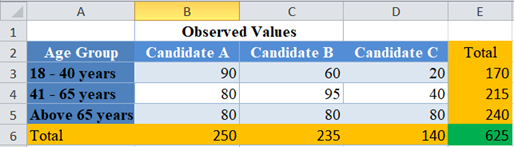Next, calculate the expected value for each cell as shown below. The expected value is given by column total times the row total divided by the grand total.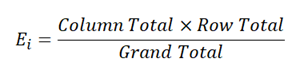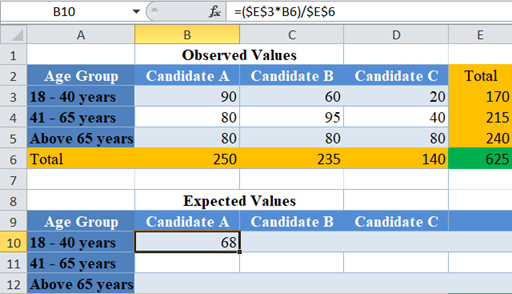Complete the expected values for the other cells and calculate the totals for each row and each column.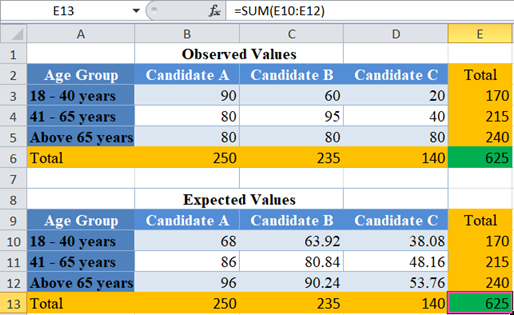Notice that the totals for the observed values are the same as the totals for the expected values. This is always the same if you performed your calculations accurately.

Now, perform the Chi-Square Test (Calculate the p-value) using the CHISQ.TEST Function.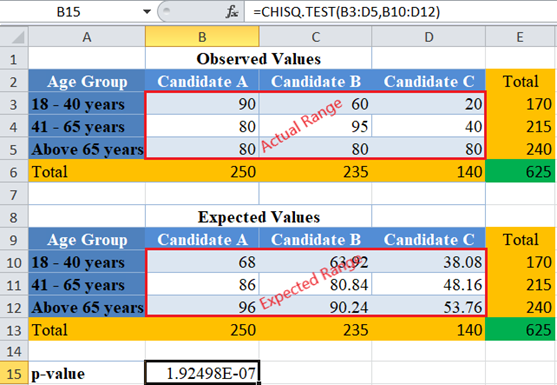The p-value here is  1.92498 x 10  -7  which is a very small number and is less than our significant level of 5% or 0.05. Therefore, we conclude that there is an association/relationship between the ages of voters and their preference for the candidates.

### CHISQ.INV.RT Function

The CHISQ.INV.RT Function returns the inverse of the right-tailed probability (the critical value) of the chi-squared distribution which is used to make a conclusion whether there is an association between the variables.

If the observed Chi-Square value is greater than the critical value, it means that there is an association/relationship between the variables in the dataset.

### Easy Excel Automation### CHISQ.INV.RT Function Syntax

``=CHISQ.INV.RT(probability (significant level), degree of freedom)``

### How to Use CHISQ.INV.RT Function

Background: A political researcher wants to know if there is a relationship between the age distribution of voters and their preference for the three leading candidates for an election. He surveyed voters of different ages on their preferred candidates and presented the result in the table below. Test, at a 5% significant level, whether there is an association between the age distribution of voters and their choice of candidate.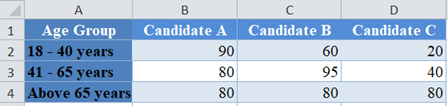Follow the steps listed earlier to calculate up to Expected Values for each of the cells.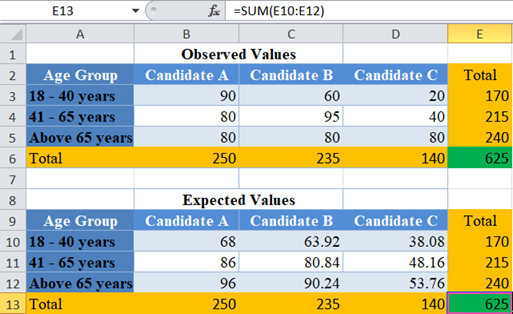Next, calculate the observed Chi-Square value for each cell by dividing the square of the difference between each actual value and expected value by the expected value and adding up all the results as shown below.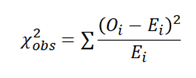where  is the observed value for each cell, and  is the expected value for each cell.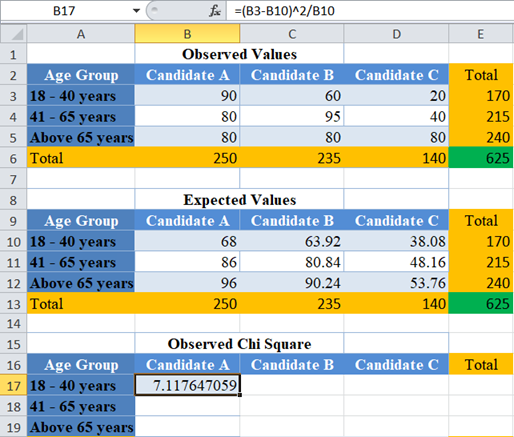Complete the rest of the cells and calculate the total for each row and the grand total.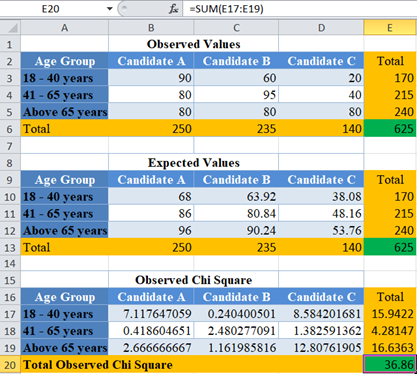Now, calculate the Chi-Square critical value using the CHISQ.INV.RT Function.

The significance level serves as the probability. Here, the significance level is 0.05. The degree of freedom (df) is given by the product of row (r) – 1 and column (c) – 1. Here there are three rows and three columns, that is degree of freedom =(3-1)(3-1) = (2)(2) = 4.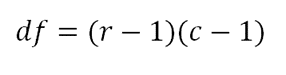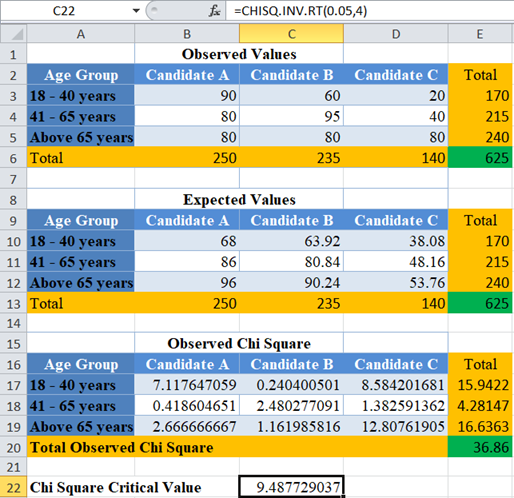Here, the observed Chi-Square is 36.86 and the critical value is 9.487729037. Clearly, the observed Chi-Square is greater than the critical value; hence, we conclude that there is an association/relationship between the ages of voters and their preference for the candidates.

# Chi-Square Test of Independence in Google Sheets

Chi-Square test of independence can be calculated using similar methods in Google Sheets.

You can use the CHISQ.TEST Function the same way as in Excel.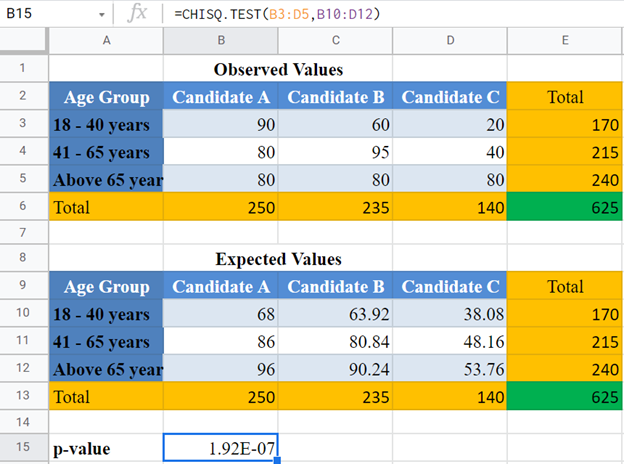Note: CHISQ.TEST and CHITEST Functions perform exactly the same function. So, you can use any of them and achieve the same result.

Or use the CHISQ.INV.RT Function:

## Common Errors – CHISQ.TEST & CHISQ.INV.RT Functions

Some of the errors you may encounter when using the CHISQ.TEST and CHISQ.INV.RT Functions.

• TEST Function returns #N/A error value when:
• The number of data points for the actual range is different from the number of data points (cells) for the expected range.
• If there is only one data point (one cell)
• INV.RT Function returns #VALUE error value when either the probability or the degree of freedom arguments is not a number.
• INV.RT Function returns #NUM error value when:
• The probability is greater than 1 or less than 0.
• The degree of freedom is less than 1 or greater than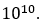.
• INV.RT Function expects the degree of freedom argument to be an integer, when not an integer, the function returns the value for the integer part. That is, CHISQ.INV.RT Function will return the same value for the degrees of freedom values of 3, 3.2, 3.8, etc.